# Singular Value Decomposition and Image Processing

The singular value decomposition (SVD) is a factorization of a real or complex matrix. It has many useful applications in signal processing and statistics.

### Singular Value Decomposition

SVD is the factorization of a $m \times n$ matrix $Y$ into three matrices as:

With:

• $U$ is an $m\times n$ orthogonal matrix
• $V$ is an $n\times n$ orthogonal matrix
• $D$ is an $n\times n$ diagonal matrix

In R The result of svd(X) is actually a list of three components named d, u and v, such that Y = U %*% D %*% t(V).

</embed>

#### Example

• we can reconstruct Y

### Image processing

• Load the image and convert it to a greyscale:• Apply SVD to get U, V, and D
• Plot the magnitude of the singular values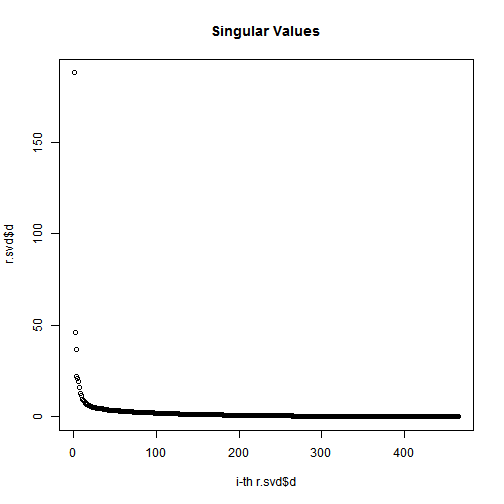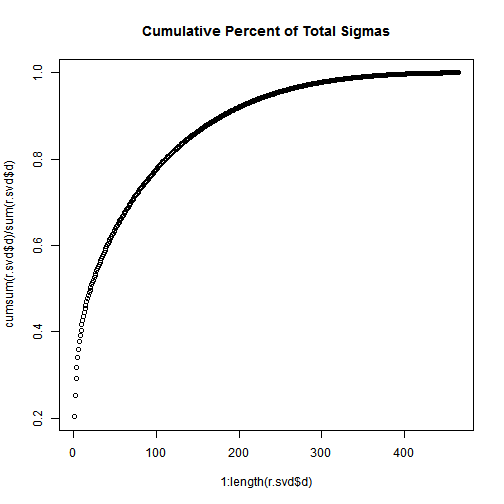Not that, the total of the first n singular values divided by the sum of all the singular values is the percentage of “information” that those singular values contain. If we want to keep 90% of the information, we just need to compute sums of singular values until we reach 90% of the sum, and discard the rest of the singular values.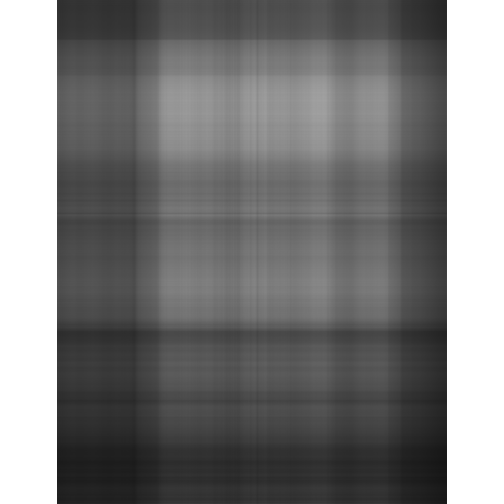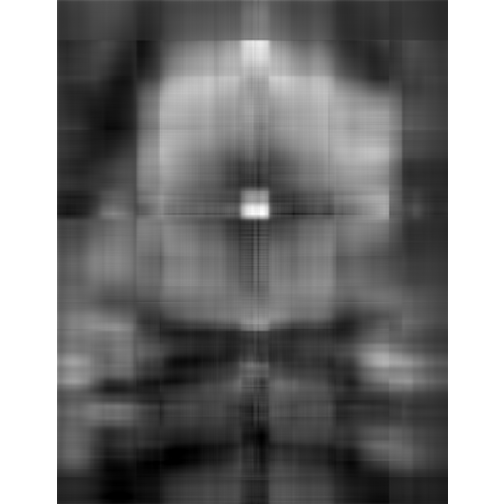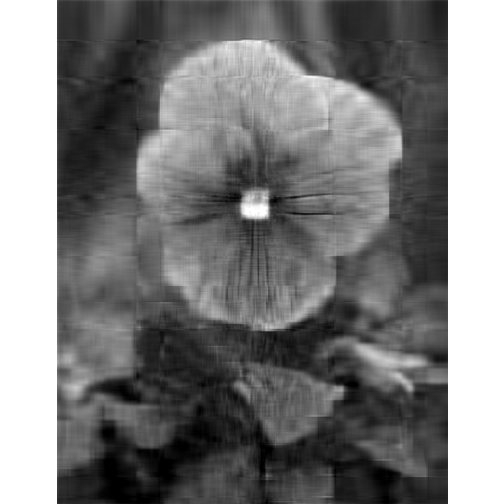### Image Compression with the SVD

Here we continue to show how the SVD can be used for image compression (as we have seen above).

• Original image• Singluar Value k = 1• Singluar Value k = 5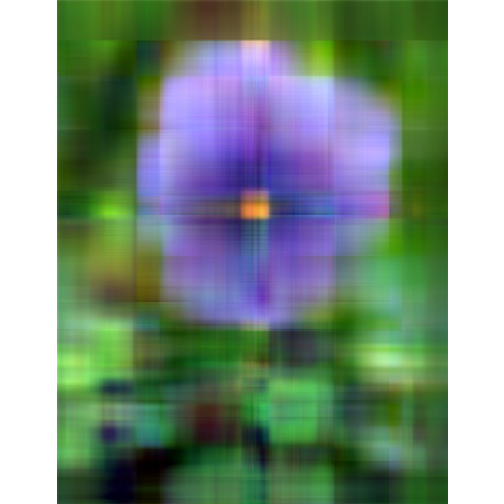• Singluar Value k = 20• Singluar Value k = 50• Singluar Value k = 100• Analysis

With only 10% of the real data we are able to create a very good approximation of the real data.

If you liked this post, you can share it with your followers or follow me on Twitter!